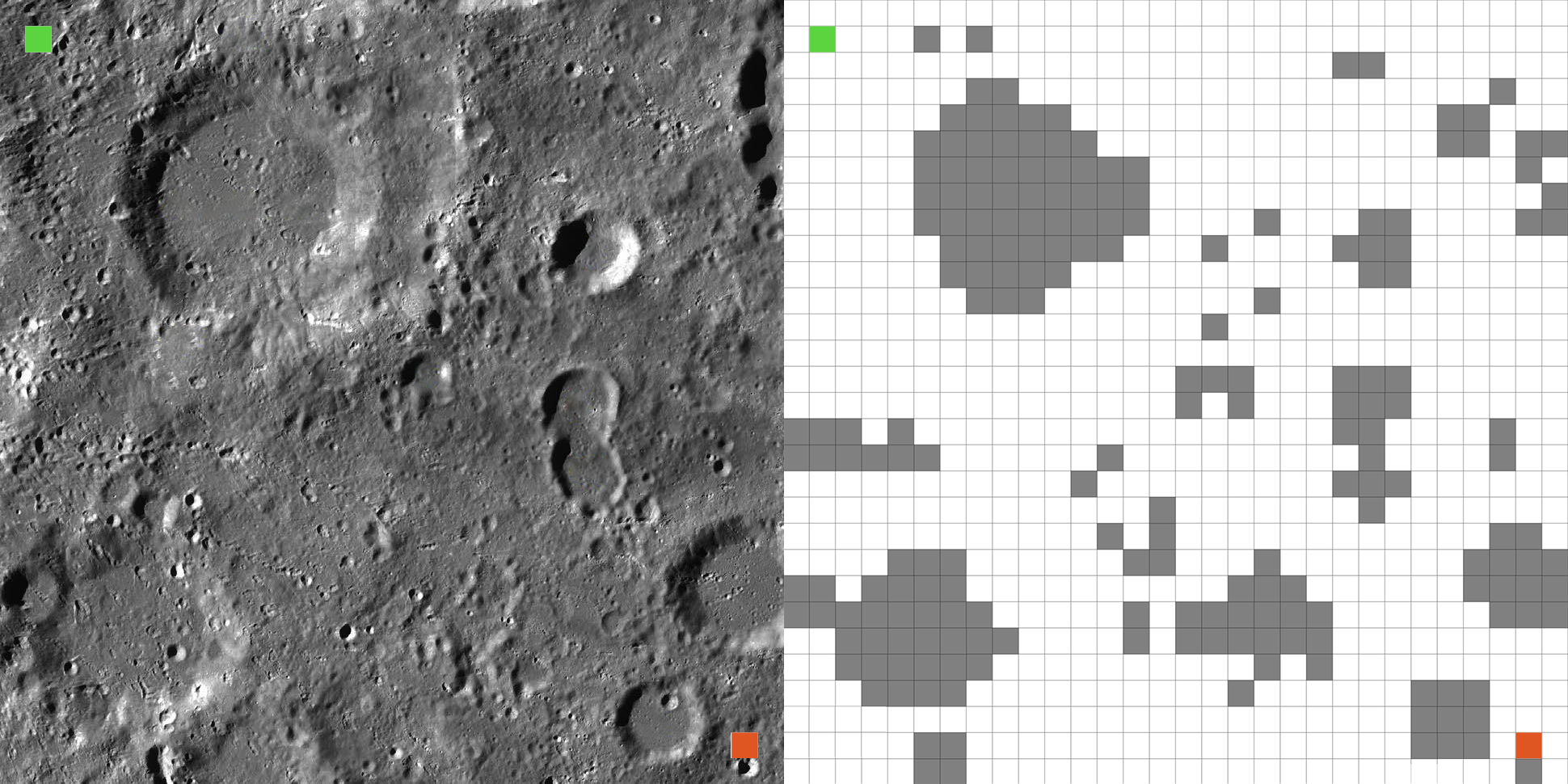# Case Study

To study path finding and the multi-threading problem, we will take the case of an autonomous rover that has to go from point A to point B with several obstacles on its path that it cannot cross. The following image represents the region on which the rover must evolve. It must go from point A (top left), to point B (bottom right). To visualize the places where the rover can or cannot pass, we have divided the map in 900 zones, and we have indicated to the rover if it can pass or not (image on the right).Image representing on the left, the region in which the rover evolves, and on the right, the simplified region.

# Pathfinding solutions

In programming, there are in the majority of cases, several solutions to respond to the same problem. Pathfinding is one of these problems. For path searching, one can use the Dijkstra algorithm, the Breadth-First Search (BFS), the Depth-First Search (DFS), Greedy or the A* algorithm.

Before you want to do multi-threading, you must first find the most optimal solution with a single thread.

We will study only very briefly the algorithms of Dijkstra, Greedy and A*.

## Dijkstra

Dijkstra’s algorithm tries to find the shortest path from the starting(root) node to every node, hence we can get the shortest path from the starting node to the goal.

Trying to solve this problem with the Dijkstra algorithm gives the following result:

We have in dark blue, the boxes where an operation has been performed, and in light blue, the places left in the list. For the resolution of the Dijkstra algorithm, we have performed 1391 operations were perfomed in 2.8550 ms.

## Greedy (Best-Frist-Search)

Greedy is an algorithm which makes a choice based on educated guesses(heuristics) at each stage. The node with shortest heuristic distance from the goal node will be explored next.

Trying to solve this problem with the Greedy algorithm gives the following result:

For the resolution of the Greedy algorithm, we have performed 161 operations were perfomed in 1.5200 ms.

## A* (A-star)

A* is a combination of Dijkstra and Greedy. It uses distance from the root node plus heuristics distance to the goal. The algorithm terminates when we find the goal node.

Trying to solve this problem with the A* algorithm gives the following result:

For the resolution of the A* algorithm, we have performed 170 operations were perfomed in 0.8250 ms.

## Conclusion

Even though the Greedy solution has 9 fewer operations, the most optimal solution is solution A* because it is almost twice as fast. We will therefore use this solution for the implementation of multi-threading.

The advantage of multi-threading is that sections of code can be executed simultaneously, which speeds up the execution time by using the available processing resources. This allows the processor to remain responsive without having to divide long sections of code into several potentially costly operations.

The major disadvantage of multi-threading is that you have to organize the code correctly, because it is difficult to debug multi-threaded code.

# How to run the A* solution in multi-threaded mode?

The most obvious solution, would be to use several threads to calculate simultaneously all the squares around the rover position, and thus find the most optimal one.

The other solution would be to run the same algorithm twice, where the starting point would be for one the starting point of the rover, and for the other, the end point. In both cases, the point of arrival of one will be the current point of the other. This implies a continuous sharing of resources between the two threads, and the implementation of semaphores, to make sure that the problem is solved correctly.

## How does it work ?

The execution of the program is done according to the following scheme:

To explain the program, we have 2 threads working at the same time. Let’s take thread A as an example:

Thread A will execute the algorithm A* with the current position of the Rover as the starting point, and the current position of the algorithm resolution of thread B as the end point. Once it has found the most optimal position where it should go, it signals to thread B that it has finished its calculation, and therefore waits until it has finished. It will execute the operation as many times as necessary until thread A and thread B have an area to calculate at the same time.

So we understand that the current position of the two threads is shared data.

With this logic, the following result is obtained: